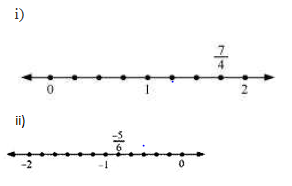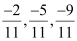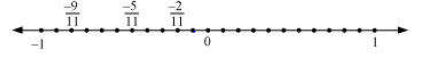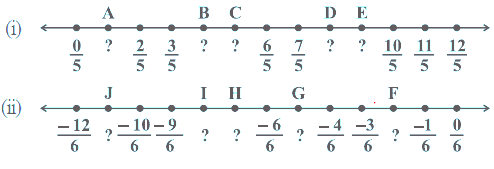# Rational numbers class 8 ncert Solutions Chapter 1 Exercise 1.2

In this page we have Rational numbers class 8 ncert Solutions Chapter 1 Exercise 1.2 .This Exercise includes questions on Rational numbers representation on Number line and how to find the rational numbers between two rational numbers. Hope you like them and do not forget to like , social share and comment at the end of the page.

## Rational numbers class 8 ncert Solutions Chapter 1 Exercise 1.2

Question 1
Represent these numbers on the number line.
1. 7/4
2. -5/6Question  2  Represent on the number line.Question 3
Write five rational numbers which are smaller than 2.
2 can be written as $\frac {14}{7}$
So the five rational number smaller than
$\frac {8}{7}, \frac {9}{7},\frac {10}{7},\frac {11}{7},\frac {12}{7}$

Question 4
Find ten rational numbers between $-\frac {2}{5}$ and $\frac {1}{2}$
Creating same denominator of both the numbers
$-\frac {2}{5} = - \frac {8}{20}$
$\frac {1}{2}= \frac {10}{20}$
So ten rational numbers will be
$-\frac {7}{20},-\frac {6}{20},- \frac {5}{20} ,- \frac {4}{20} ,-\frac {3}{20},- \frac {2}{20},- \frac {1}{20}, 0 ,\frac {1}{20},\frac {2}{20}$
Question 5
Find five rational numbers between
(i) $\frac {2}{3}$ and $\frac {4}{5}$
(ii) $- \frac {3}{2}$ and $\frac {5}{3}$
(iii) $\frac {1}{4}$ and $\frac {1}{2}$
1. Creating same denominator $\frac {2}{3} = \frac {30}{45}$
$\frac {4}{5}= \frac {36}{45}$
So five rational number will be
$\frac {31}{45}, \frac {32}{45}, \frac {33}{46}, \frac {34}{45},\frac {35}{45}$
2. Creating same denominator $- \frac {3}{2}= - \frac {9}{6}$
$\frac {5}{3}= \frac {10}{6}$
So five rational number will be
$-\frac {8}{6},- \frac {7}{6},-1,- \frac {5}{6},- \frac {4}{6}$
3. Creating same denominator
$\frac {1}{4}= \frac {8}{32}$
$\frac {1}{2}= \frac {16}{32}$
So five rational number will be
$\frac {10}{32}, \frac {11}{32}, \frac {12}{32},\frac {13}{32}, \frac {14}{32}$
Question 6
Write five rational numbers greater than - 2.
-2 can be written as -14/7
So the five rational number smaller than
-8/7,-9/7,-10/7,-11/7,-12/7
Question 7
Find ten rational numbers between $\frac {3}{5}$ and $\frac {3}{4}$
$\frac {3}{5}= \frac {48}{80}$
$\frac {3}{4}=\frac {60}{80}$
So ten rational numbers are
49/80, 50/80, 51/80, 52/80, 53/80, 54/80, 55/80, 56/80, 57/80, 58/80

## Try these NCERT book Solutions

Write the rational number for each point labelled with a letteri. 1/5, 4/5,5/5,8/5,9/5
ii. -11/6, -8/6,-7/6, -5/6,-2/6

## Summary

1. Rational numbers class 8 ncert Solutions Chapter 1 Exercise 1.2 has been prepared by Expert with utmost care. If you find any mistake.Please do provide feedback on mail. You can download the solutions as PDF in the below Link also
2. This chapter 1 has total 2 Exercise 1.1 and 1.2. This is the last exercise in the chapter.You can explore previous exercise of this chapter by clicking the link below